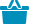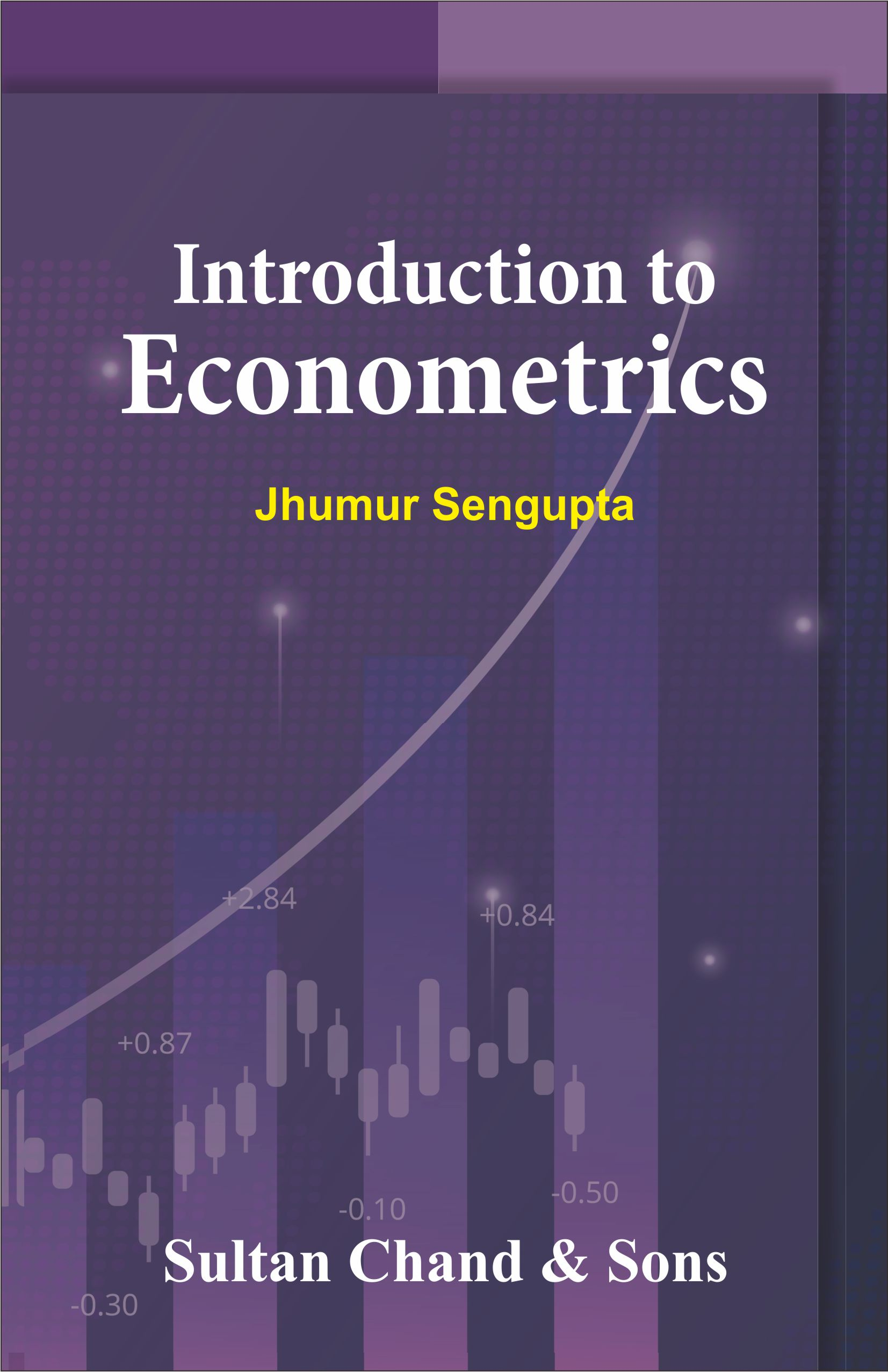Cart

# Introduction to Econometrics#### Quick Overview

Authored By

Publisher: Sultan Chand & Sons

Publishing Year: 2023

Size (mm): 210.00 x 145.00

ISBN: 978-93-91820-31-2

Page nos.: xvi + 176

MRP: 295.00

Subject: Economics

₹295.00

The book is intended for the Core Course on “Introductory Econometrics” for Economics Honours students at the Undergraduate level according to the National Education Policy (NEP), 2020 and Choice Based Credit System syllabus. All the UGC-recognized Universities are the potential users of the book. In addition, the book covers a part of the UGC NET Syllabus. Students and researchers who want to learn basic Econometric theory will find the book very useful. The book addresses the basic theories of Econometrics in a clear and lucid manner.

# Salient Fetures

• The book covers topics including regression models, parameter estimation techniques, properties of the estimators, statistical testing and model specification problems in detail.
• Elementary concepts of statistics have been provided in Chapter 1 of the book. For ease of understanding, chapters on advanced topics are covered in the later part of the book.
• Statistical and mathematical derivations are used in the book in a thorough manner for the students and researchers who do not have any exposure to the course Econometrics.
• Each chapter contains several examples and exercise problems illustrating the applications of econometric theories.
• Some of the examples and exercise problems have been taken from the UGC NET Examination, Examinations at several Universities and Competitive Examinations.
• Every effort has been made to explain the basic theories in a simple way for easy understanding of the subject.
• A discussion on Computer Packages STATA and R is given in the Appendix Section.

• Nature and Scope of Econometrics

• Estimation of Classical Linear Regression Model

• Properties of Least Square Estimators

• Statistical Inference in Linear Regression Model

• Data Problems & Violations of Classical Assumptions

• Specification Analysis

ISBN13: 978-93-91820-31-2

Weight: 0.00

Edition: First Edition

Language: English

Title Code: 1280

#### Author

Authored By : Sengupta Jhumur (Dr)